# Pass-By Value and Pass-By Reference in JavaScript

Challenge Inside! : Find out where you stand! Try quiz, solve problems & win rewards!
Learn via video courseJavaScript Course With Certification: Unlocking the Power of JavaScript
By Mrinal BhattacharyaFree
4.8Enrolled: 1000JavaScript Course With Certification: Unlocking the Power of JavaScript
Mrinal BhattacharyaFree
4.8
Enrolled: 1000

## Overview

In JavaScript, when a function is called, the arguments can be passed in two ways, either Pass by value or Pass by reference (address). Primitive data types such as string, number, null, undefined, and boolean, are passed by value while non-primitive data types such as objects, arrays, and functions are passed by reference in Javascript.

## Prerequisites

To understand Pass by Value and Pass by Reference in JavaScript you first need to have an understanding of the following topics:

Note: In JavaScript, primitive values are stored on the stack, while non-primitive values are stored in a heap.

## Pass by Value in JavaScript

Pass by value in JavaScript means that a copy of the actual parameter’s value is made in memory i.e., a new memory allocation is done, and all the changes are made in that new value (i.e., copied value). The original value and the copied value are independent of each other as they both have a different space in memory i.e., on changing the value inside the function, the variable outside the function is not affected.

In simple language, we can understand it as, in a pass-by value, the function receives a copy of the variable, which is independent of the originally passed variable.

Pass by value in JavaScript requires more space as the functions get a copy of the actual content therefore, a new variable is created in the memory.

In this concept, equals operator plays a big role. When we create a variable, the equals operator notices whether you are assigning that variable a primitive or non-primitive value and then works accordingly.

Note: When we use the = operator, there is a function call (behind the scenes) where pass by value (or reference) in JavaScript is done.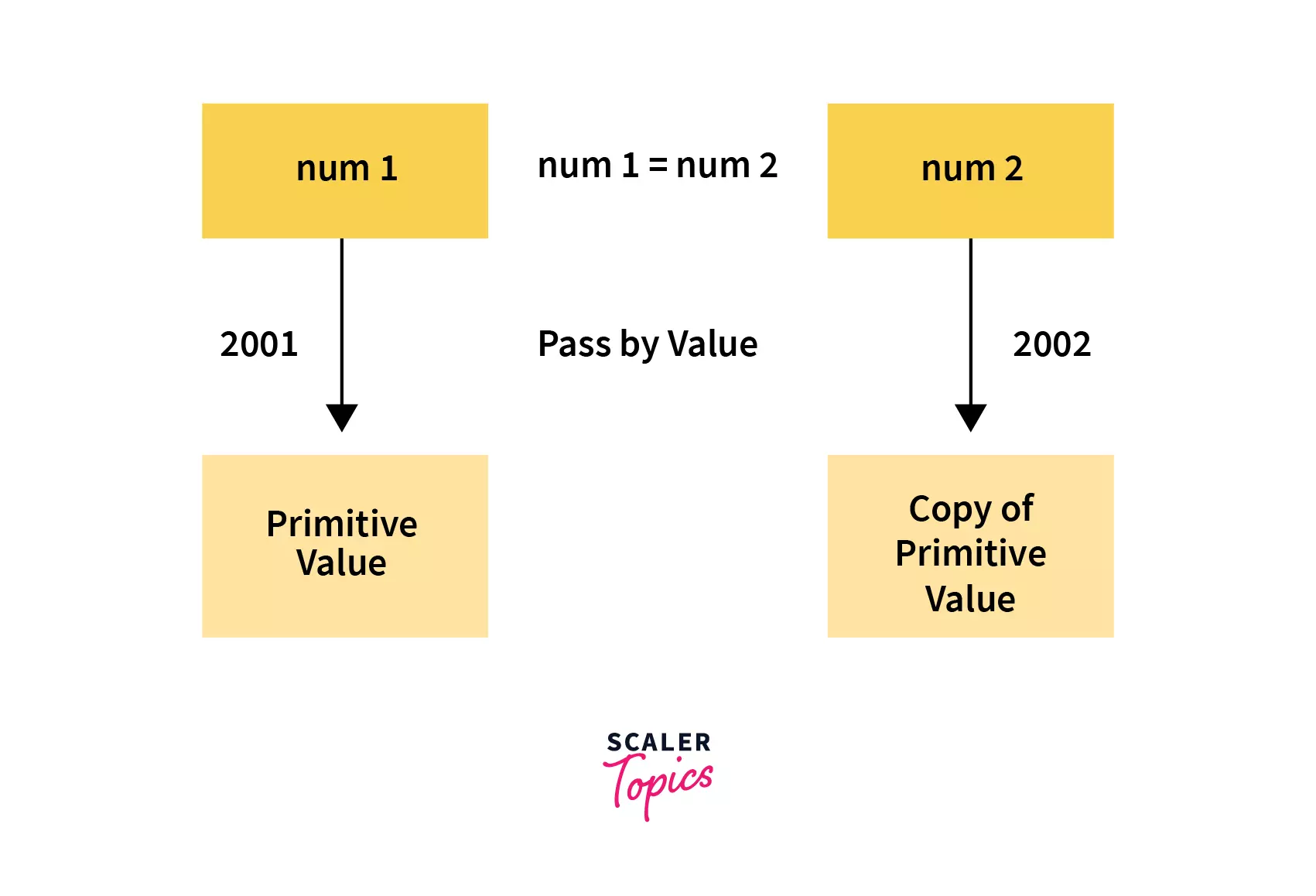When we assign a variable a primitive value, the equals operator sets up a space (location/address) in the memory (let's say at address 2001) to store the data of that variable (num1) to that address.

Now, when we created a new variable num2 (ley's say at address 2002) and assign it the value of the previous variable num1, the equals operator creates NEW SPACE in memory which is independent of the previous variable num1 with address 2001 and places its copy (num1) in the newly created variable space in the memory. Thus, this copies the value of the original variable, num1, into two separate spots in memory (with addresses 2001 and 2002).

Here, we have assigned num1 a value of 70. This creates a space in memory by the name num1 and addresses 2001 (assumption). When we create a variable num2 and assign it the value of num1, then equals operator notices that we’re dealing with a primitive values thus it creates a NEW SPACE in memory with address 2002 and assigns it a copy of num1's value, i.e. 70. Now we can see that both the variables have different spaces in the memory, and both have a value of 70.

Now, if we change the value of num1, then num2 will have no effect as it has its own separate space in memory and now it has nothing to do with the value of num2 as they both have different spaces (address) in memory.

Let's understand this better by another example:

From the above code, we can see that the function multiplication takes an argument and changes its value.

Then we have declared a variable num, with a value of 30.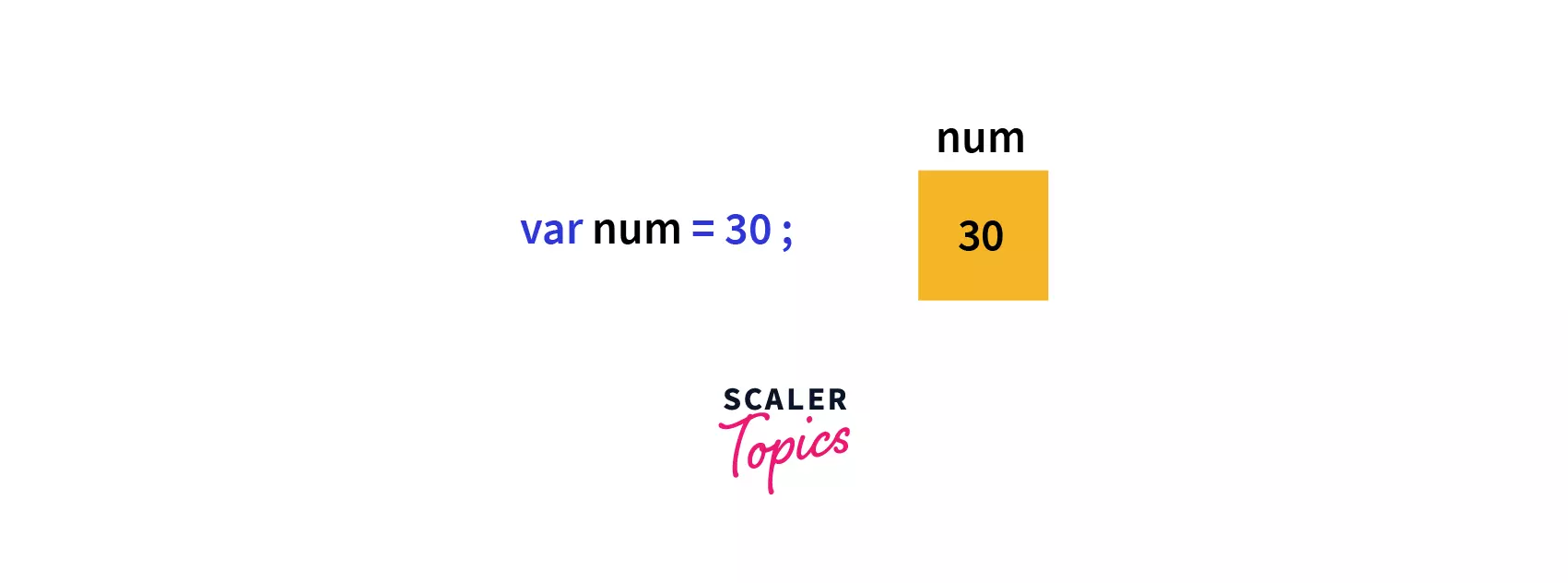After that, we passed the variable num to the multiplication function. Javascript automatically copies the value of variable num to variable tmp. So, here tmp is a new variable that is allocated a new space in the memory and is independent of num.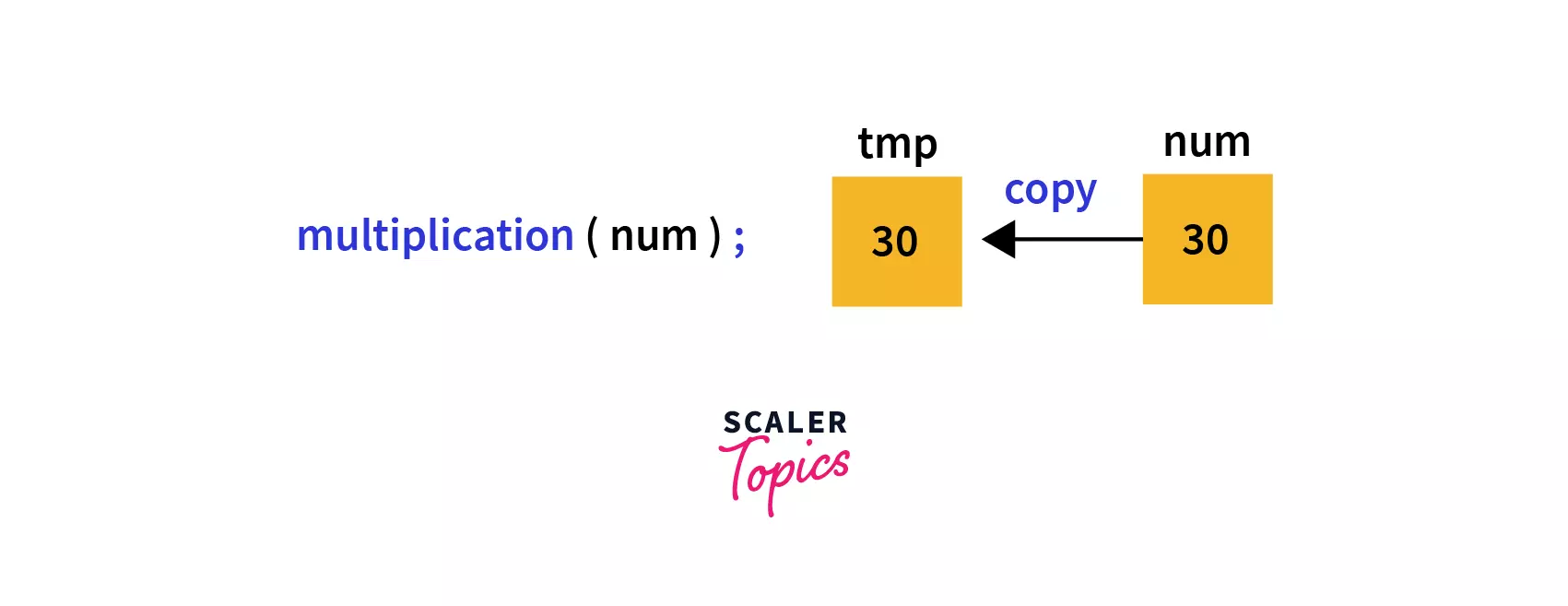Now all the changes made by the function multiplication are done directly to the variable tmp; thus the value of num remains unaffected.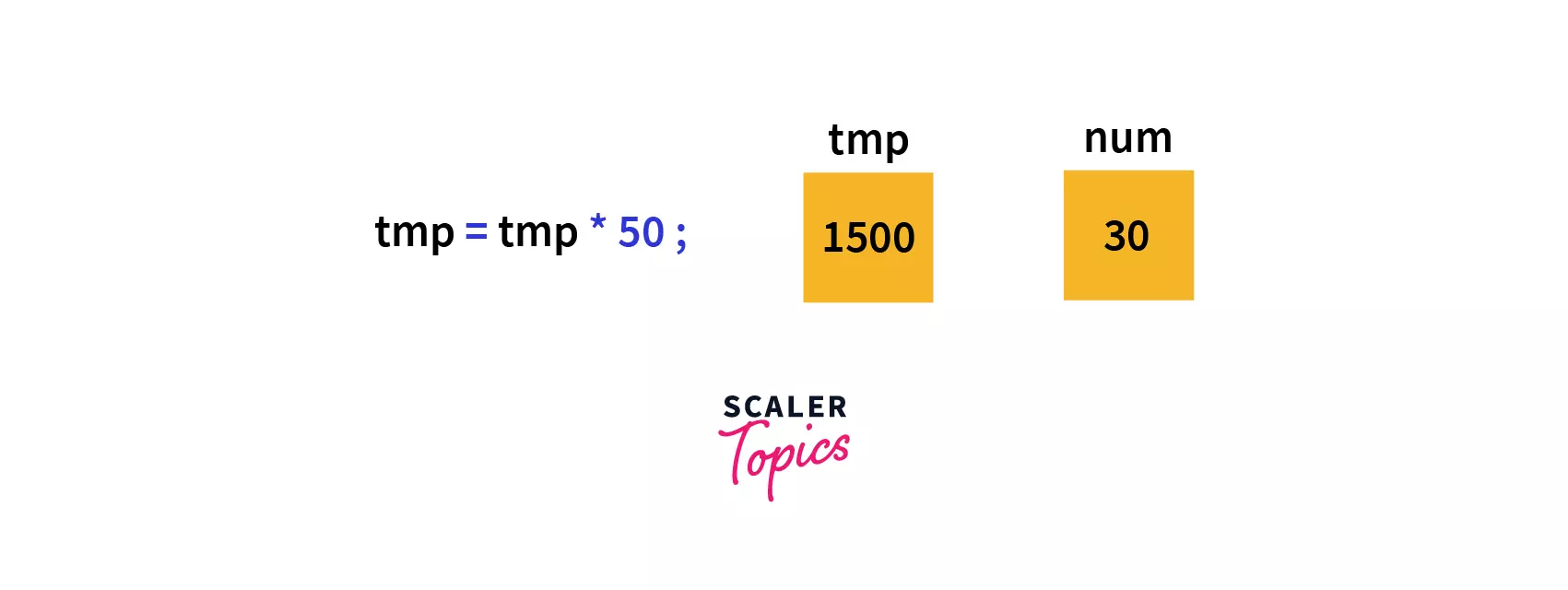This happens because a separate copy of variable num is created in the memory named tmp with initial value 30, which after calculation becomes 1500.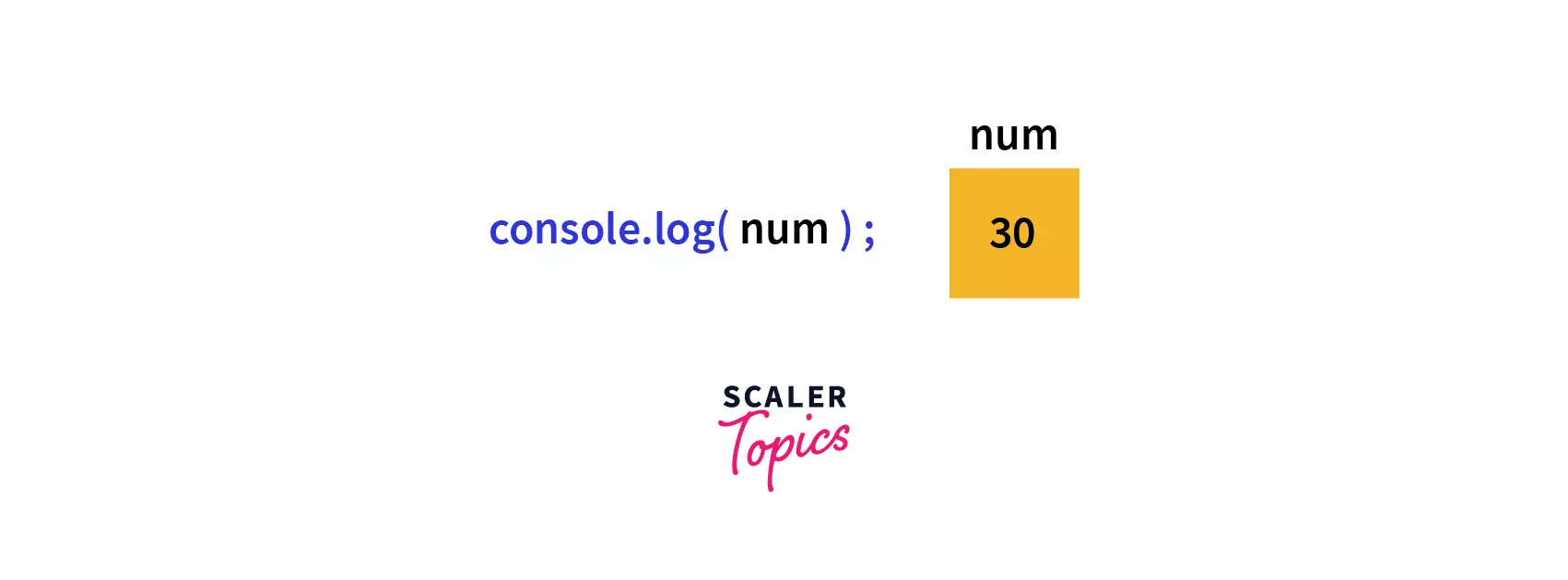tmp and num have no link with each other i.e., they are independent of each other.

## Pass by Reference in JavaScript

Unlike pass by value in JavaScript, pass by reference in JavaScript does not create a new space in the memory, instead, we pass the reference/address of the actual parameter, which means the function can access the original value of the variable. Thus, if we change the value of the variable inside the function, then the original value also gets changed.

It does not create a copy, instead, it works on the original variable, so all the changes made inside the function affect the original variable as well.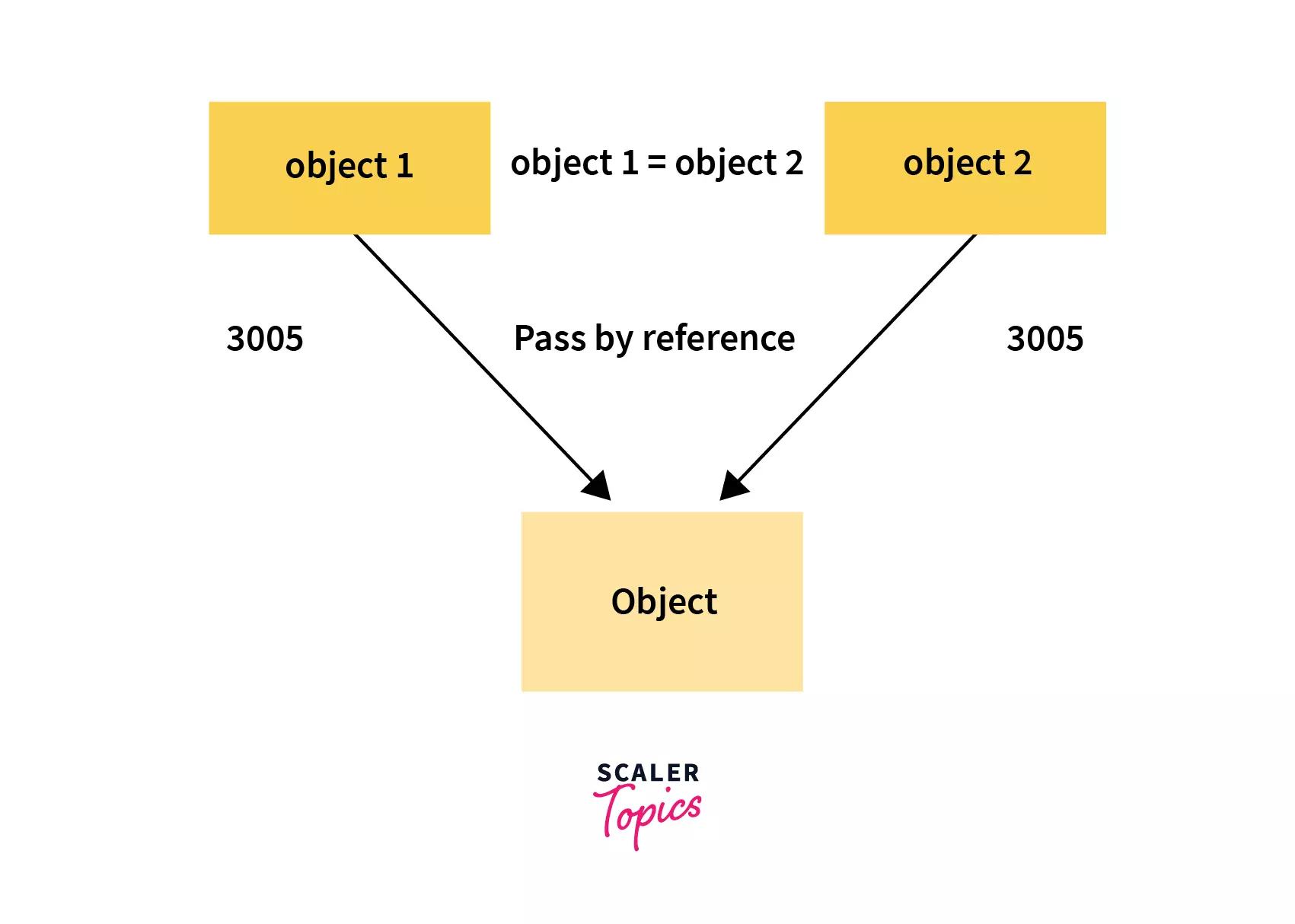Unlike pass-by value in JavaScript, here, when the equal operator identifies that the variable obj1 is set equal to an object, it creates a new memory space and points obj1 to 3005(address assumption). Now, when we create a new variable, obj2 and assign it to the value of obj1 the equals operator identifies that we are dealing with non-primitive data types; thus, it points to the same address that obj1 points to. Thus we can see that no new memory space is created instead, both the variables are pointing to the same address that obj1 was pointing to.

In the above example, we have made a variable obj1 and set it equal to an object, then we have set the value of another variable obj2 equal to obj1.

As the equal operator identifies that we are dealing with non-primitive data types, so instead of creating a new memory space, it points obj2 to the same memory space that obj1 is pointed to. Thus when we change (mutate) the value of obj1, then the value of obj2 also gets changed since obj2 is also pointing to the same memory space as obj1 does.

### Pass by Reference in Object (with Function)

From the above example, we can see that on changing the value tmpObj, the value of originalObj also gets changed. The reason for this is that when we call demo and pass the object, then originalObj is passed by its reference, so the local parameter tempObj will point to the same object which we defined, i.e., the originalObj.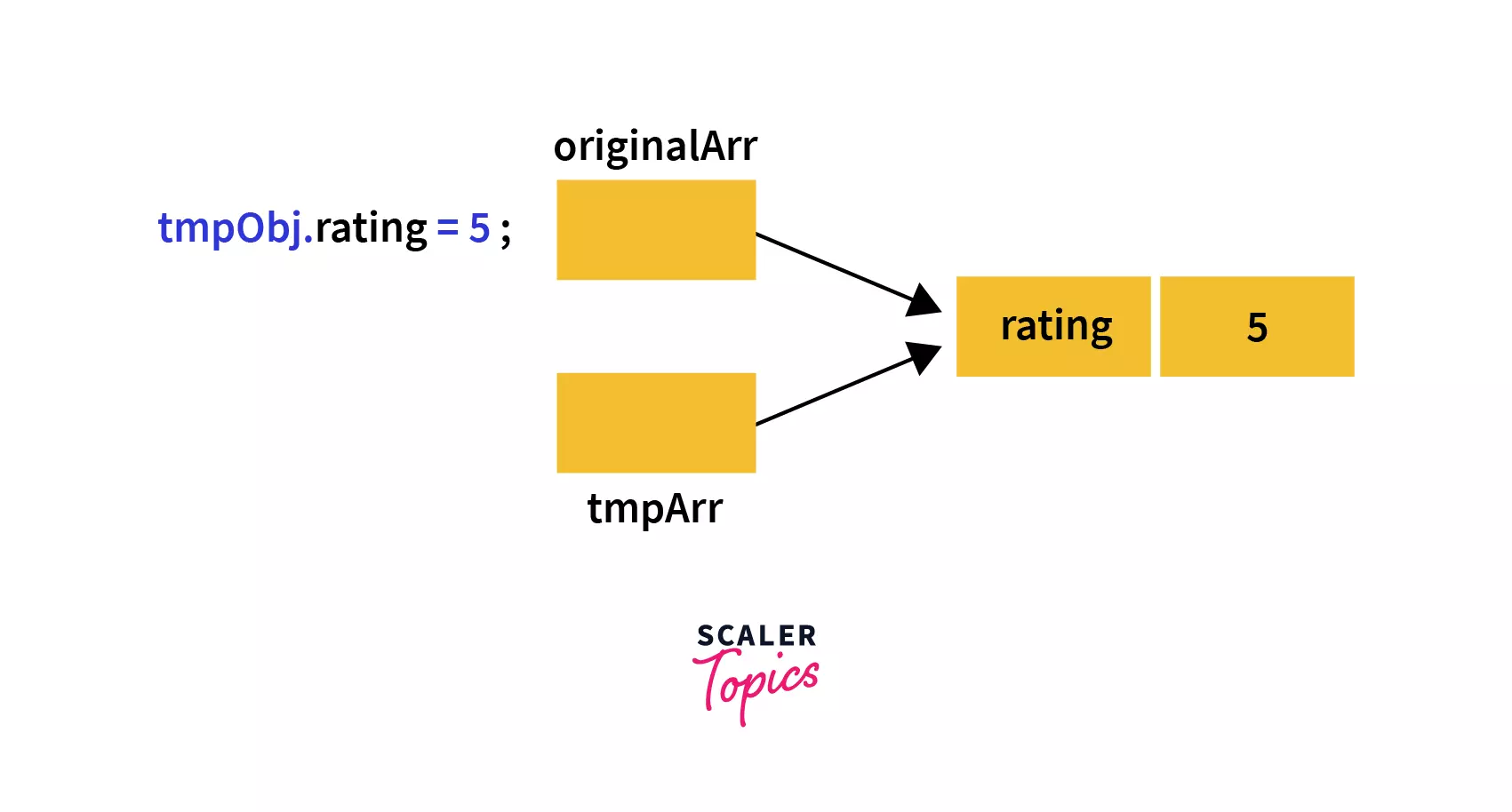So, in this case, we are not dealing with two independent copies instead, we have variables that are pointing to the same object, so, any changes made to this object will be visible to the other variable.

### Pass by Reference in an Array (with Function)

Here, when we are trying to add a new item to the array stored in tempArr, it also affects the originalArr array. This happens because there are no two separate copies of an array, we are dealing only with one array. The variable tempArr references the same array that was initialized in the variable originalArr.

This example states that, like objects, in arrays also, on changing the value of tempArr, the value of originalArr changes automatically.

Thus we can conclude by saying all the non-primitive data types interact by the reference, so when we set their values equal to each other or pass them to a function, then they all point to the same memory space (address) whenever we change one of the value, then all of the values get changes.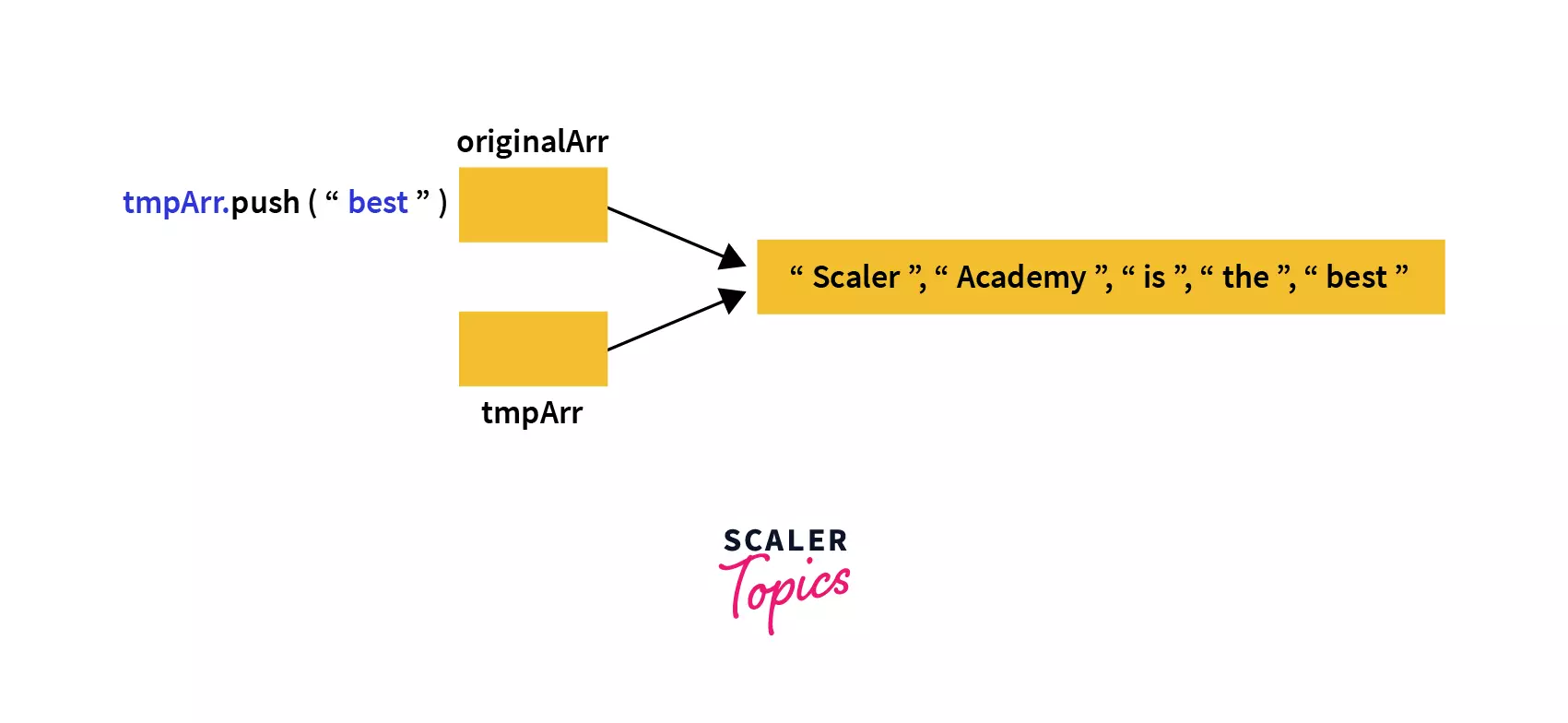## When to Use Pass by Value?

As in pass-by value in JavaScript, a new copy of the variable is created, and any changes made in the new variable are independent of the original variable, so it is useful when we want to keep track of the initial variable and don't want to lose its value.

## When to Use Pass by Reference?

When we are passing arguments of large size, it is better to use pass-by-reference in JavaScript as no separate copy is made in the called function, so memory is not wasted, and hence the program is more efficient.

## Conclusion

• In JavaScript, we have value types, also called primitives, and reference types (non-primitives) which are objects.
• Primitives are number, string, boolean, symbol, undefined, and null, whereas, Non-primitives are objects, functions, and arrays.
• In pass-by value in JavaScript, a copy of the original variable is created, so any changes made to the copied variable do not affect the original variable.
• In pass-by reference in JavaScript, we pass the reference of the actual parameter. No copy is created in the memory.